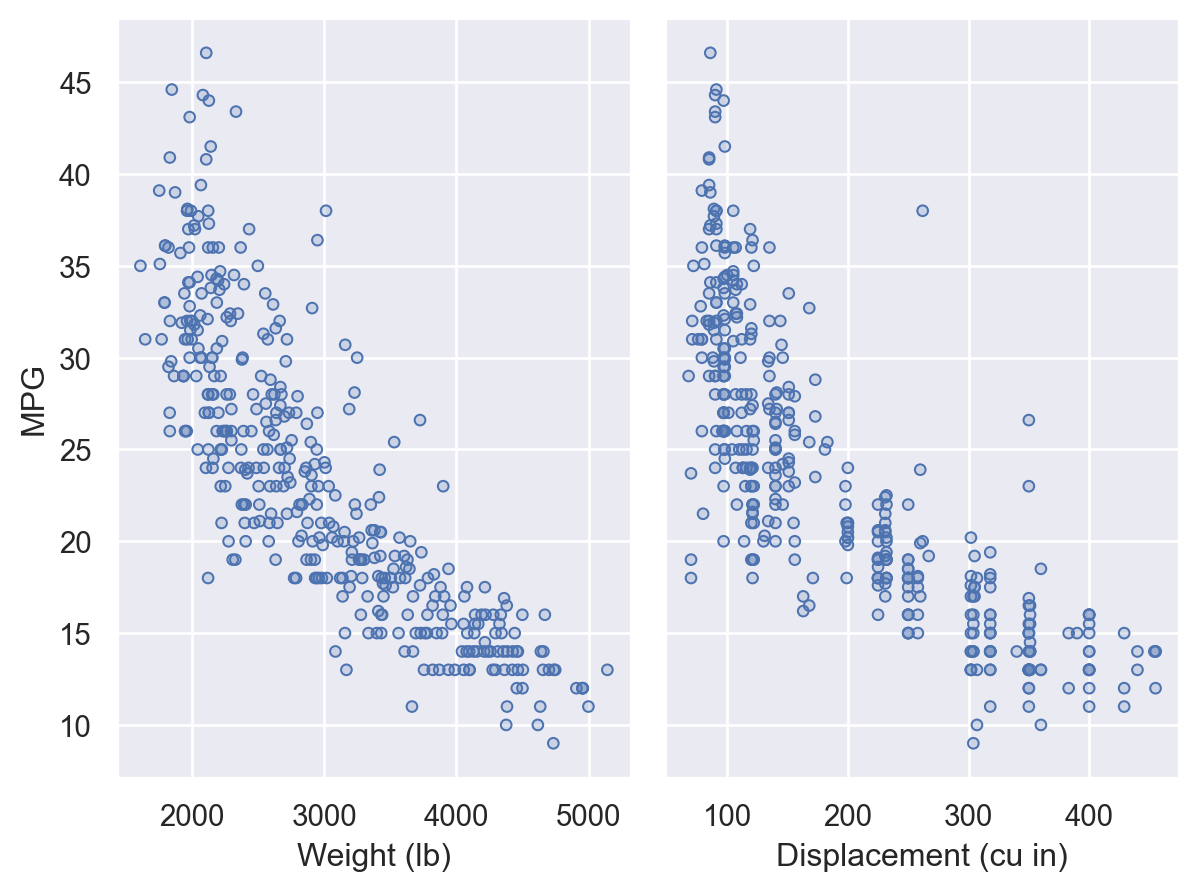# seaborn.objects.Plot.pair#

Plot.pair(x=None, y=None, wrap=None, cross=True)#

Produce subplots by pairing multiple `x` and/or `y` variables.

Parameters:
x, ysequence(s) of data vectors or identifiers

Variables that will define the grid of subplots.

wrapint

When using only `x` or `y`, “wrap” subplots across a two-dimensional grid with this many columns (when using `x`) or rows (when using `y`).

crossbool

When False, zip the `x` and `y` lists such that the first subplot gets the first pair, the second gets the second pair, etc. Otherwise, create a two-dimensional grid from the cartesian product of the lists.

Examples

Plot one dependent variable against multiple independent variables by assigning `y` and pairing on `x`:

```(
so.Plot(mpg, y="acceleration")
.pair(x=["displacement", "weight"])
)
```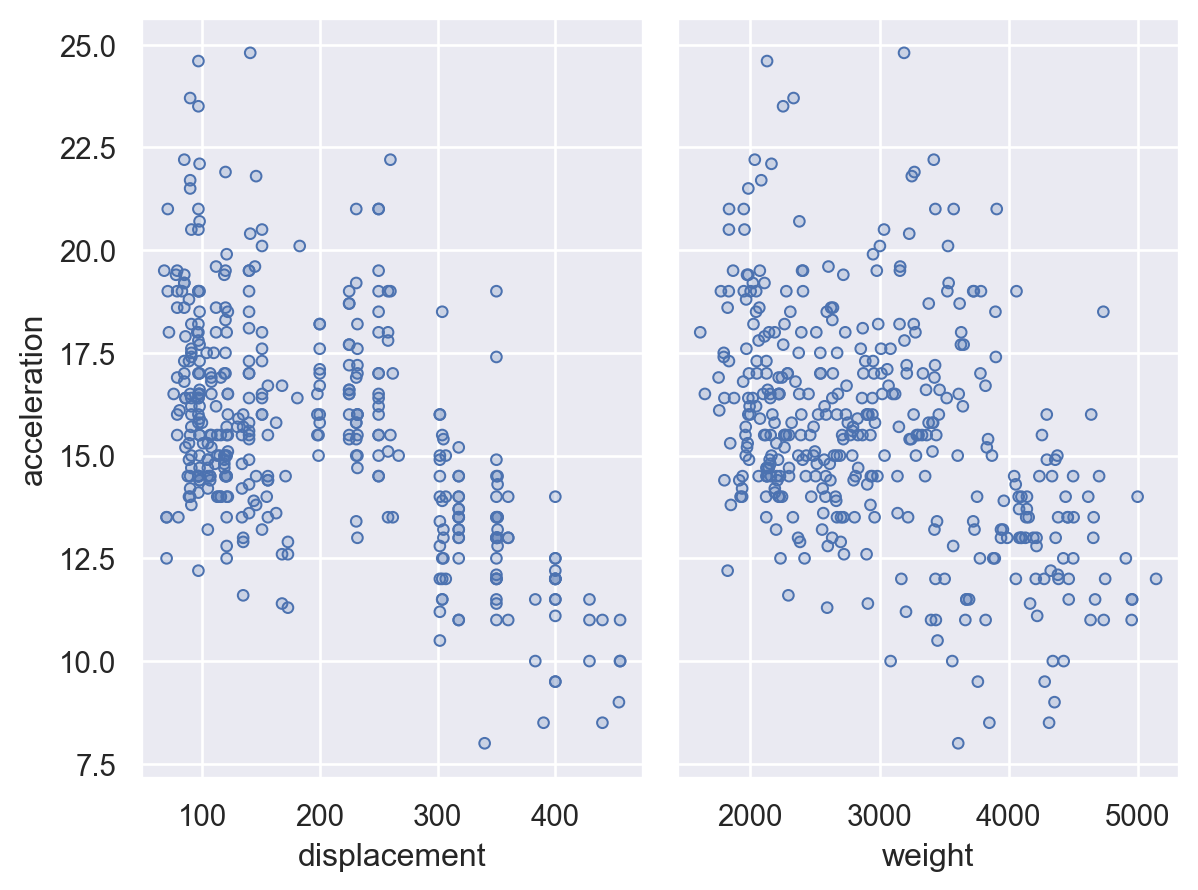Show multiple pairwise relationships by passing lists to both `x` and `y`:

```(
so.Plot(mpg)
.pair(x=["displacement", "weight"], y=["horsepower", "acceleration"])
)
```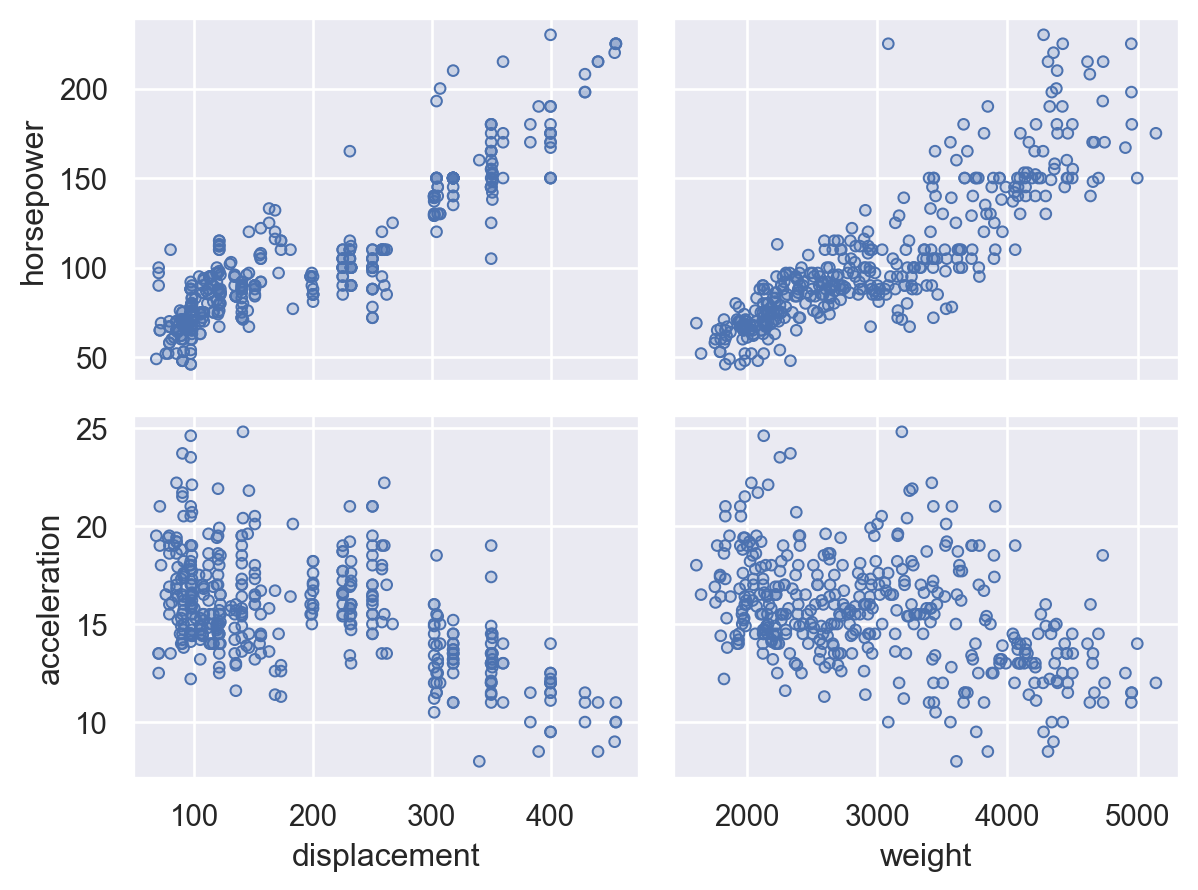When providing lists for both `x` and `y`, pass `cross=False` to pair each position in the list rather than showing all pairwise relationships:

```(
so.Plot(mpg)
.pair(
x=["weight", "acceleration"],
y=["displacement", "horsepower"],
cross=False,
)
)
```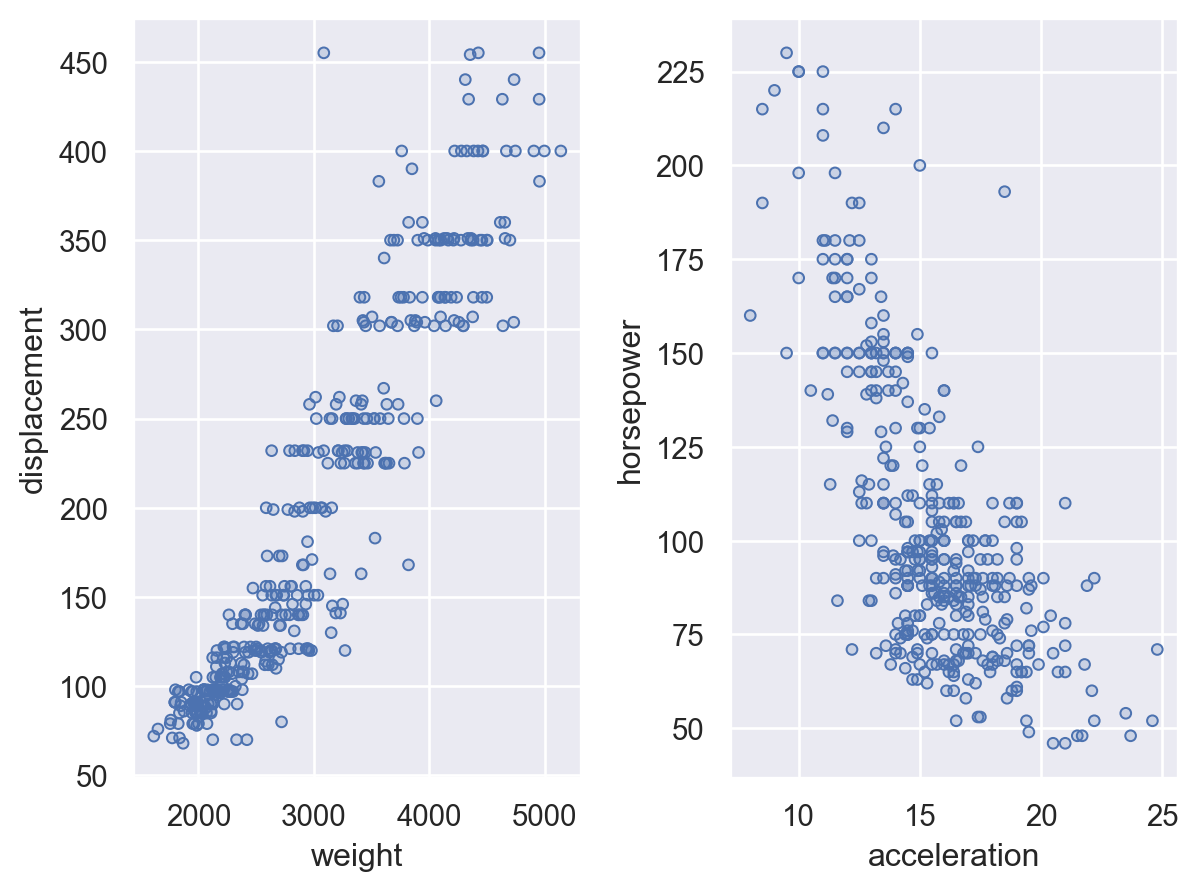When plotting against several `x` or `y` variables, it is possible to `wrap` the subplots to produce a two-dimensional grid:

```(
so.Plot(mpg, y="mpg")
.pair(x=["displacement", "weight", "horsepower", "cylinders"], wrap=2)
)
```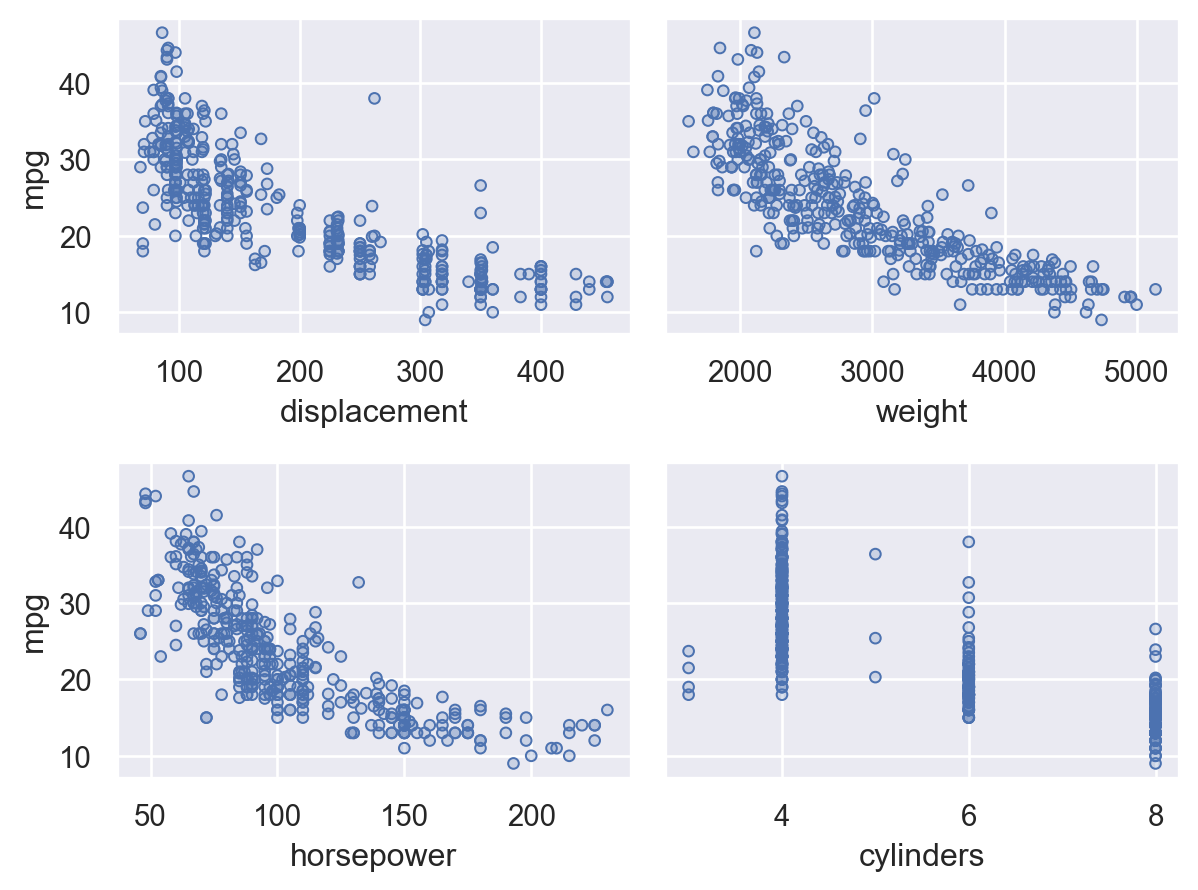Pairing can be combined with faceting, either pairing on `y` and faceting on `col` or pairing on `x` and faceting on `row`:

```(
so.Plot(mpg, x="weight")
.pair(y=["horsepower", "acceleration"])
.facet(col="origin")
)
```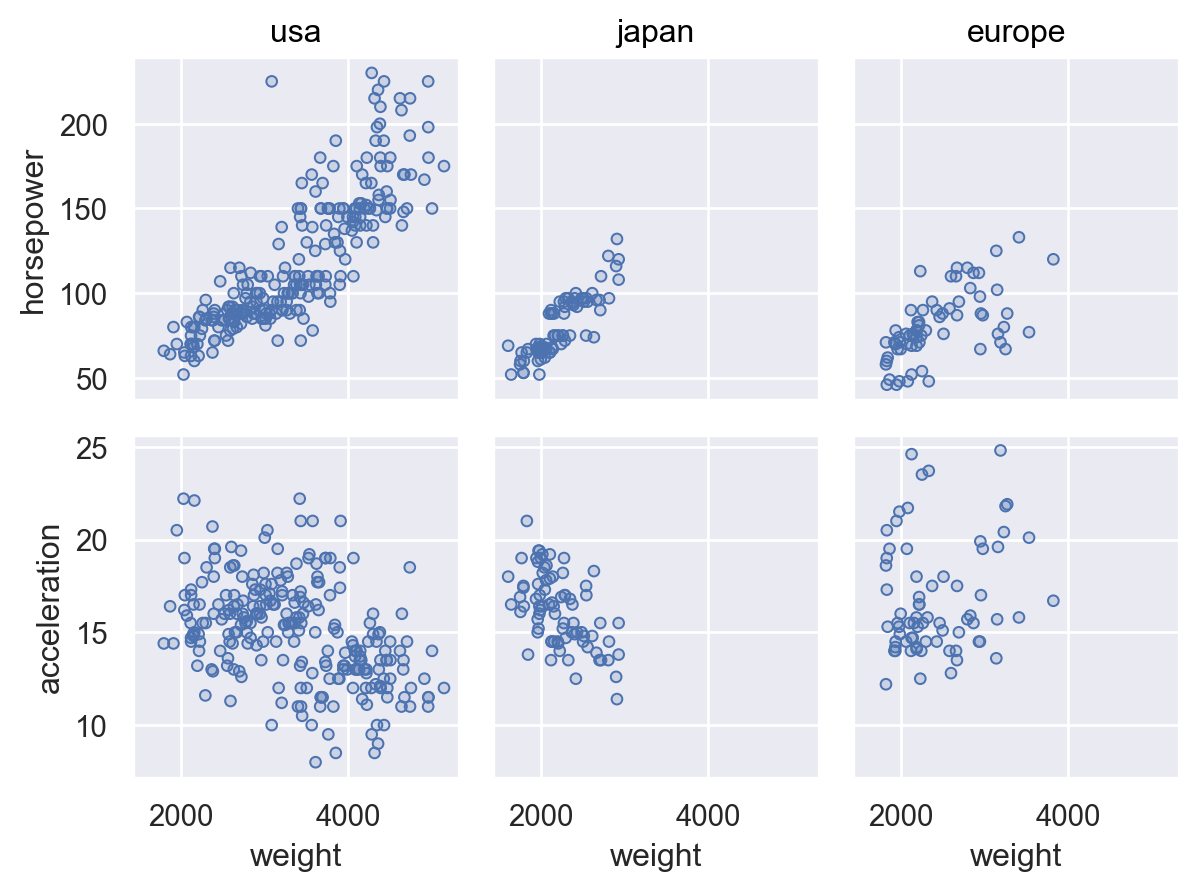While typically convenient to assign pairing variables as references to the common `data`, it’s also possible to pass a list of vectors:

```(
so.Plot(mpg["weight"])
.pair(y=[mpg["horsepower"], mpg["acceleration"]])
)
```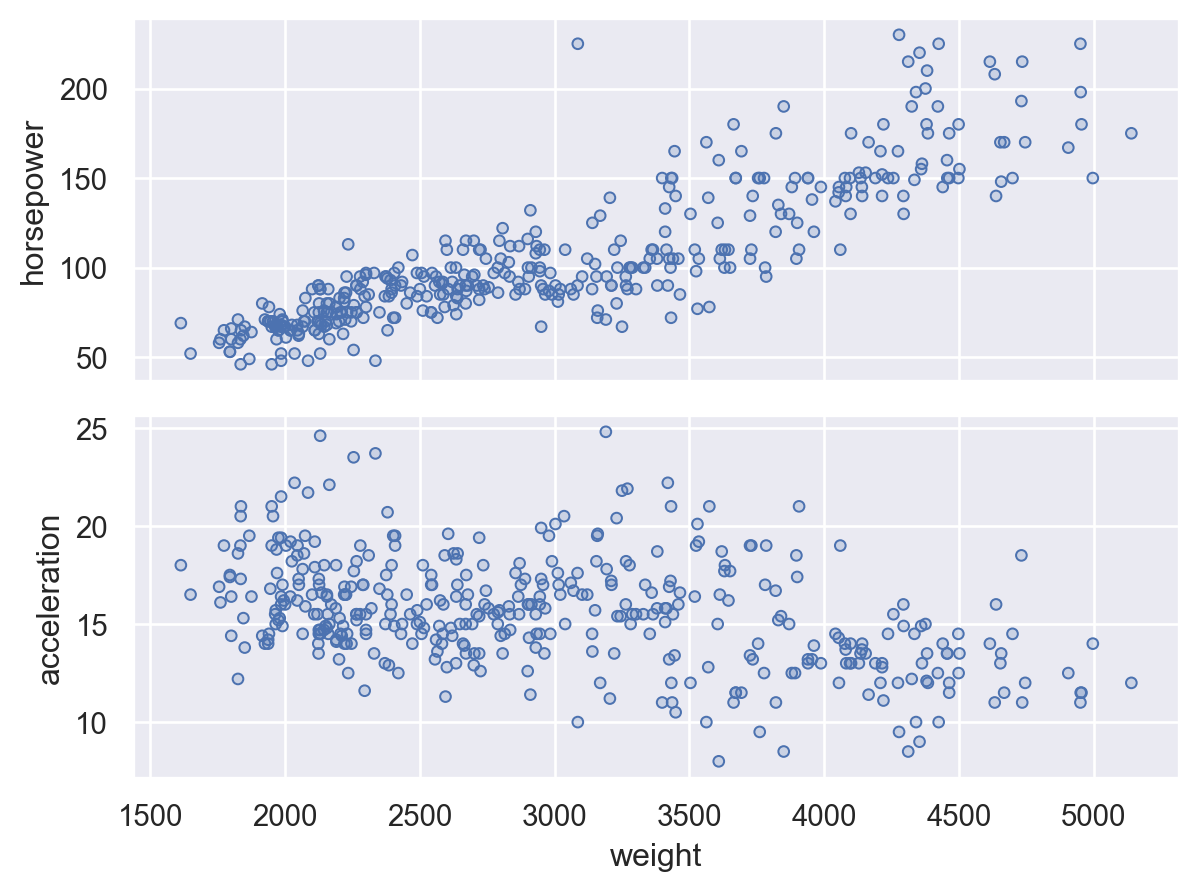When customizing the plot through methods like `Plot.label()`, `Plot.limit()`, or `Plot.scale()`, you can refer to the individual coordinate variables as `x0`, `x1`, etc.:

```(
so.Plot(mpg, y="mpg")
.pair(x=["weight", "displacement"])
.label(x0="Weight (lb)", x1="Displacement (cu in)", y="MPG")